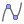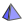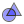# Descărcați aplicațiile GeoGebra

## Aplicații GeoGebra offline gratuite pentru iOS, Android, Windows, Mac, Chromebook și Linux

• ### Calculator Suite

Explore functions, solve equations, construct geometric shapes and 3D objects.

•### Calculator de grafice

Graph functions, investigate equations, and plot data with our free graphing app

•### 3D Calculator

Graph 3D functions, plot surfaces and do 3D geometry with our free 3D Grapher

•### Geometrie

Construct circles, angles, transformations and more with our free geometry tool

• ### GeoGebra Clasic 6

Apps bundle including free tools for geometry, spreadsheet, probability, and CAS

• ### Calculator CAS

Solve equations, expand and factor expressions, find derivatives and integrals

• ### GeoGebra Clasic 5

Apps bundle including free tools for geometry, spreadsheet, probability, and CAS